Next: Flux Freezing Up: Basic Equations of Magnetohydrodynamics Previous: Basic Equations of Magnetohydrodynamics   Contents

# Magnetohydrodynamics

Here, we derive the basic equations of magnetohydrodynamics. Differences from hydrodynamics are the Lorentz force in the equation of motion and the induction equation of magnetic field. The Lorentz force is written as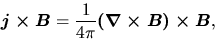(B.1)

where we used the Ampere's law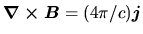and the displacement current is ignored. Thus, the equatin of motion becomes as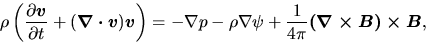(B.2)

in the Eulerian form.

The Faraday's law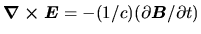and the Ohm's law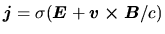(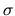is the electric conductivity) lead the induction equation of the magnetic field as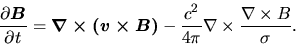(B.3)

The factor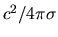is the coefficient of magnetic diffusibility. When we assume the electric conductivity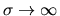, diffusive MHD equation, equation(B.3), reduces to the ideal MHD equation as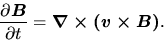(B.4)

SubsectionsNext: Flux Freezing Up: Basic Equations of Magnetohydrodynamics Previous: Basic Equations of Magnetohydrodynamics   Contents
Kohji Tomisaka 2007-07-08